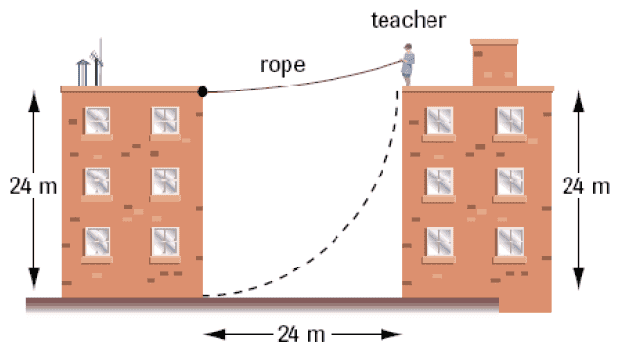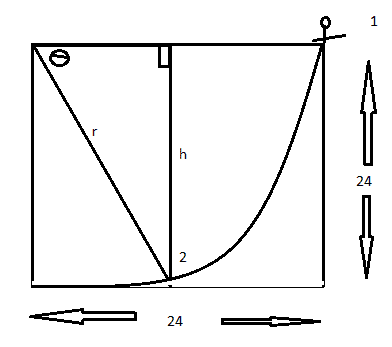# Circular Motion Problem w/ Conservation of Energy

mxwtt

## Homework Statement

Your favourite physics teacher who is late for class attempts to swing from the roof of a 24m long rope as shown in the picture. The teacher starts from rest (Ek=0) with the rope horizontal, but the rope will break if the tension force in it is twice the weight of the teacher. How high is the swinging physics teacher above the ground when the rope breaks?
(Hint: Use the conservation of energy)Ek=(1/2)mv^2
Eg=mgh
Fc=(mv^2)/r

## The Attempt at a Solution

My diagram:Eg = mgh
= m(9,8)(24)
= 235.2m
Ek = (1/2)mv^2
= mv^2
(because it says that the rope will break if the tension force in it is twice the weight of the teacher)
then i equate these.
235.2m = mv^2
235.2 = v^2
v = 15.3 m/s.
from here i have no idea what to do. i think i might have to use Fc=(mv^2)/r, but i not sure how.
thanks in advance for you help.